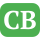CppBuzz.com

# C++ program to find sum of n numbers using function

``````#include <stdio.h>
#include <conio.h>

int sum (int number);

int main()
{
int no;

printf("\n How many numbers? : ");
scanf("%d", &no);

printf("\n Sum of the %d numbers is : %d ",no,sum(no));

return 0;

}
int sum(int number){